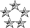## Need help To Understand Pigeonhole Principle

Mathematics for Computing
NeoPosts: 2642
Joined: Wed Jul 15, 2009 2:07 am
Location: Colombo

### Re: Need help To Understand Pigeonhole Principle

For the theorems explained in Herath's post, you need to know about Floor and Ceiling of numbers. I have added a little post on that. What are ceiling and floor in mathematics?

Generalizations of the pigeonhole principle
A generalized version of this principle states that, if N discrete objects are to be allocated to k containers, then at least one container must hold no fewer than ⌈N / k⌉ objects. Similarly, at least one container must hold no more than ⌊N/k⌋ objects.

First question.
k = 4, We need to find N.
⌈N / 4⌉ = 4
N should be minimum 13 to be ⌈13 / 4⌉ = ⌈3.25⌉ = 4

To verify, ⌈12 / 4⌉ = ⌈3⌉ = 3, So 12 is not going to work.
Second part,
There are 7 days in a week. Then we have 7 pigeonholes. So the answer should be 29.
I'll give a little explanation.

k = 7, We need to find N.
⌈N / 7⌉ = 5
N should be minimum 29 to be ⌈29 / 7⌉ = ⌈4.14⌉ = 5

To verify, ⌈28 / 7⌉ = ⌈4⌉ = 4, So 28 is not going to work.
Third part,
There are 12 months in a year. So we have 12 pigeonholes. In order to have at least 2 students born in the same month we should have 13 students in the class.
k = 12, We need to find N.
⌈N / 12⌉ = 2
N should be minimum 13 to be ⌈13 / 12⌉ = ⌈1.083⌉ = 2

To verify, ⌈12 / 12⌉ = ⌈1⌉ = 1, So 12 is not going to work.